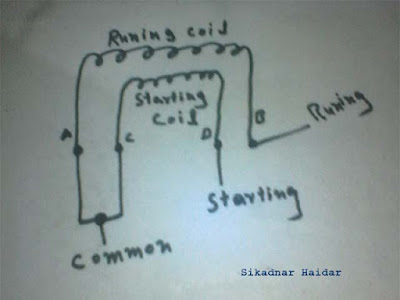# What is Start Run Common In Single Phase Motor

Mostly in our daily life single phase motor are use then 3 phase motor. Stand fan, Ceiling fan, refrigerator compressor etc are all single phase motor. When ever we talk about the single phase or one phase motor we here about the start run and common. But we don't know that what is start run and common. This post will help you that what is this in one phase motors.

## Start Run Common Diagram :

In a single phase motor have two types of coil and we called him running and starting coil or winding. and these two coil have one starting point and one ending point.

So the connection between running winding wire and starting winding point is called common, we called him common because this point is related with both winding coils. And the other running winding wire is called run terminal or Run connection of motor and same the other starting coil point is called start connection. The below start run common diagram help you for better understanding.start run common diagram

In the above example diagram, i shown  two coil symbols, the running coil starting point is A and ending point is B.The starting coil starting point is C and ending point is D. So if we connect A and C then this will called the common because this point is connected with both coils. The ending point of running coil is called Run and end point of starting called the Start.
Note A capacitor will be connect between the run and start terminals.

Message :
Now i hope you understood that what is start, run and common in a single phase motor winding. Now if you have any question regarding this article then you can ask me by using the below comments section.

1.2.